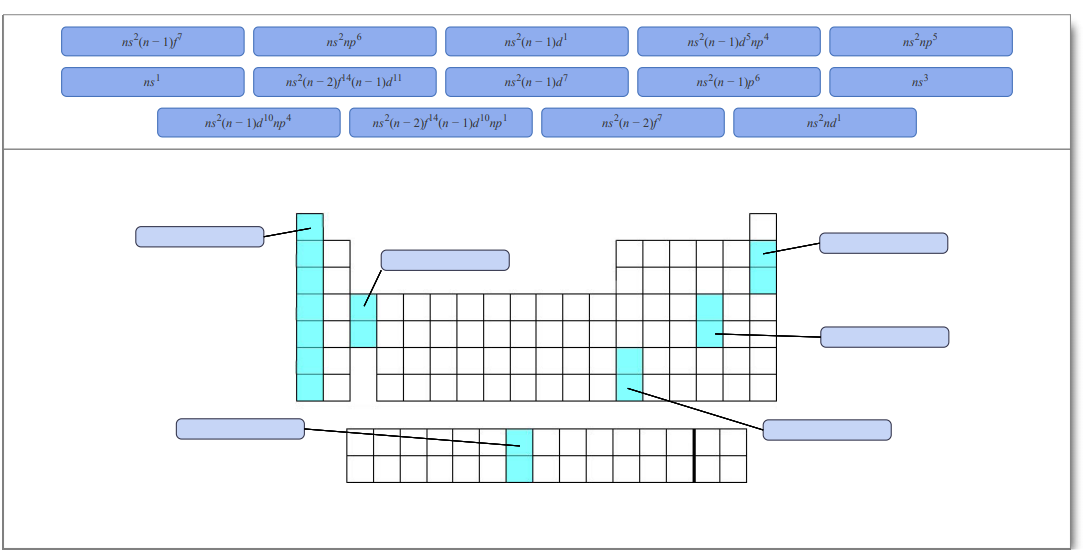# Problem: Identify the general outer electron configuration for each group of elements shown in this periodic table outline.The periodic table lists all known elements arranged by atomic number. Atomic number is the nuclear charge, the number of protons in the nucleus of an an atom of a particular element. For a neutral atom, the number of protons is equal to the number of electrons. Each column of the table, called a group, contains elements with the same number of valence electrons that are in different quantum levels. Each row of the table, called a period, contains elements with differing numbers of valence electrons that are in the same principal quantum level. The four main blocks of the table (s, p, d, and f) contain elements whose highest energy electrons have the same azimuthal quantum number (ℓ).

###### FREE Expert SolutionView Complete Written Solution
###### Problem Details

Identify the general outer electron configuration for each group of elements shown in this periodic table outline.The periodic table lists all known elements arranged by atomic number. Atomic number is the nuclear charge, the number of protons in the nucleus of an an atom of a particular element. For a neutral atom, the number of protons is equal to the number of electrons. Each column of the table, called a group, contains elements with the same number of valence electrons that are in different quantum levels. Each row of the table, called a period, contains elements with differing numbers of valence electrons that are in the same principal quantum level.

The four main blocks of the table (s, p, d, and f) contain elements whose highest energy electrons have the same azimuthal quantum number (ℓ).